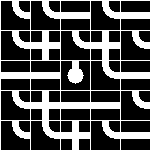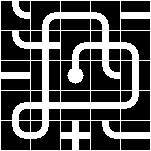Problem Statement

The pipe game is played on a grid with three kinds of pipes. The straight pipe '-' allows water to flow straight. The elbow pipe 'L' diverts water either to the left or to the right. The cross pipe '+' has the same effect as a straight pipe. Unlike the other two pipes, the cross pipe allows water to flow through it a second time in a direction perpendicular to the first pass. The water source is represented by one of: 'N', 'S', 'W' or 'E' indicating whether the water will begin flowing north, south, west or east respectively. You are allowed to rotate each pipe in the grid by 90 degree increments with the objective of maximizing the number of pipes connected to the water source.

Given a String[] pipes that represents the game grid, determine the length of the longest possible flow of water. Each pipe that the water flows through adds one to the total length. A cross pipe through which water passes twice contributes two to the overall length. The starting water source is not a pipe, does not count towards the length, and may not be rotated.

{"LL-L-",
"L+L+L",
"--NL-",
"L+--L",
"LL+L-"}This is a graphical representation of the puzzle above.

Definition

 Class: PipePuzzle Method: longest Parameters: String[] Returns: int Method signature: int longest(String[] pipes) (be sure your method is public)

Notes

-In each grid, south is the direction of increasing index within pipes, and north that of decreasing index. East is the direction of increasing index within an element of pipes, and west that of decreasing index.

Constraints

-pipes will have between 1 and 20 elements, inclusive.
-Each element of pipes will have length between 1 and 20, inclusive.
-Every element of pipes will have the same length.
-pipes will only contain the characters ('-', 'L', '+', 'N', 'S', 'E', 'W').
-pipes will have exactly one water source.
-pipes will contain between 0 and 20 elbow 'L' pipes, inclusive.

Examples

0)

 {"LL-L-", "L+L+L", "--NL-", "L+--L", "LL+L-"}
Returns: 191)

 {"ELLL", "LLLL", "LLLL", "LLLL"}
Returns: 132)

 {"ELLLLL+", "++++++L", "L+++++L", "L+++++L", "L+++++L", "L+++++L", "+LLLLLL"}
Returns: 71
3)

 {"-+-+-+-+-+-+-+-+-+-W"}
Returns: 19
4)

 {"N"}
Returns: 0

Problem url:

http://www.topcoder.com/stat?c=problem_statement&pm=2001

Problem stats url:

http://www.topcoder.com/tc?module=ProblemDetail&rd=8007&pm=2001

HardCoder

Testers:

PabloGilberto , brett1479 , Olexiy

Problem categories:

Recursion, Search